# Two boats

The sightseeing boat sail at 25 km / h. The cruise take 6 hours. How long the another boat sails at 60 km/h?

Result

x =  2.5 h

#### Solution:Leave us a comment of example and its solution (i.e. if it is still somewhat unclear...):Be the first to comment!#### To solve this verbal math problem are needed these knowledge from mathematics:

Most natural application of trigonometry and trigonometric functions is a calculation of the triangles. Common and less common calculations of different types of triangles offers our triangle calculator. Word trigonometry comes from Greek and literally means triangle calculation.

## Next similar examples:

1. Masons1 mason casts 30.8 meters square in 8 hours. How long casts 4 masons 178 meters square?
2. Round-tripA woman works at a law firm in city A which is about 50 miles from city B. She must go to the law library in city B to get a document. Find how long it takes her to drive​ round-trip if she averages 40 mph.
3. Cars 6At 9:00 am two cars started from the same town and traveled at a rate of 35 miles per hour and the other car traveled at a rate of 40 miles per hour. After how many hours will the cars be 30 miles apart?
4. Tourist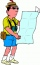A tourist walked an average speed of 3.5 km/h route in 6 hours. Calculate how many hours he would have passed at an average speed of 5.5 km/h.
5. Cenda and Pepa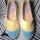Cenda and Pepa went to the event. Cenda started alone. Canda started after him for 20 minutes. How long took Cenda to catch him? Cenda traveling at 15 km/h, and Pepa traveling at 25 km/h.
6. Bob traveled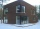Bob traveled 20 meters to Dave's house. He arrived in 3 minutes. What was his average speed for the trip.
7. Train delayThe train should be in the station for 10 minutes. It still has 32 km left. What is the expected delay if the train travels in 2 minutes 3 km except for the last section where it is 2 km in 5 minutes.
8. Average speedMichal went out of the house by car at speed 98 km/h. He came into the goal place for 270 minutes. Determine the distance between the two places.
9. StormSo far, a storm has traveled 35 miles in 1/2 hour in direction straight to observer. If it is currently 5:00 p. m. and the storm is 105 miles away from you, at what time will the storm reach you? Explain how you solved the problem.
10. Pedestrian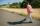Dana started at 10:00 from the point A to point B. These points are distant 12 km. Determine how fast Daniel went when the place B arrived at 11:54. Speed express in km/h.
11. Mail trainThe speed of mail train is 1370 meter per minute. Express it in miles per hour correct to three significant figure . Given that 1 meter =39.37 inches.
12. Cyclist vs car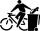Cyclist rode out of the city at 18 km/h. 1 hour 30 minutes behind him started car and caught up with the cyclist in 50 minutes. How fast was driving the car? Where (what kilometer) from the city car overtook a cyclist?
13. Greg and BillGreg is 18 years old. He is 6 less than 4 times Bill's age. How old is Bill?
14. Grandfather and grandmotherThe old mother is 5 years younger than the old father. Together they are 153 years old. How many years has each of them?
15. Diver and pressure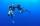Determine what pressures is exposed diver without a suit at a depth of 15 meters, with a small space suit at a depth of 25 meters and a massive suit at a depth of 70 m in water.
16. Proportion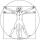write the following proportion 8 is to 64 as to 2 is to x
17. Two trains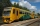From station A, the freight train traveled at a speed of 40 km/h in 9h. When he drove 15km, the fast train started from station A in the same direction at a speed of 70km/h. When will it the freight train catch up?